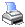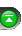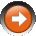﻿ Typical
 NinjaScript > Language Reference > Data > TypicalDefinition

A collection of historical bar typical prices. Typical price = (High + Low + Close) / 3.

Property Value

A DataSeries type object. Accessing this property via an index value [int barsAgo] returns a double value representing the price of the referenced bar.

Syntax

Typical
Typical[int barsAgo]

Examples

 // Current bar typical price double barTypicalPrice = Typical;   // Typical price of 10 bars ago double barTypicalPrice = Typical;   // Current bar value of a 20 period exponential moving average of typical prices double value = EMA(Typical, 20);